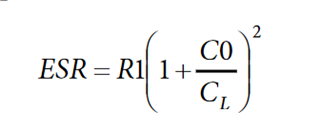# nRF52840 LFXO

I don't fully understand datasheet for LFXO.

17.4.3

CL_LFXO Load Capacitance = 12.5pF. Is this the recommended CL for external crystal?

RS_LXFO ESR = 100k. Is this the max ESR in crystal OR is this a ESR on the chip?

PD_LFXO = 1uW. Is this the recommended minimum Drive Level for crystal OR is this max outout power to crystal?

ILFXO = 0.23uA. Is this the current for silicon driving the crystal or is this the max output current to crystal?

I must be sure to not "overdrive" crystal. The crystals Drive Level I'm thinking of can be 0.1uW.

Kan anybody enlighten me because the datasheet it's not obvious to me.

Parents
• Hi,

To make sense of these specs please take a look at any 32MHz crystal datasheet.

CL_LFXO Load Capacitance = 12.5pF. Is this the recommended CL for external crystal?

This is the max recommended load capacitance given by the crystal datasheet (CL). To calculate the load capacitors value (the one connected to the crystal), C1 and C2 in our reference design, you should use the following formula:

C1 = C2 = 2CL - C_pcb - C_pin

CL: Load capacitance of the crystal (found in the crystal datasheet)

C_pcb + C_pin is approximately 4 pF.

As explained in General PCB design guidelines for nRRF52 blogpost.

RS_LXFO is the maximum equivalent series resistance, sometimes not given by the crystal manufacturer datasheet but can be calculated from:Where R1 is the motional resistance, C0 is the shunt or static capacitance of the crystal, and CL load capacitance of the crystal.

So in summary, the values from the datasheet outline the maximum values for the values on the LFXO crystal specification/datasheet you choose.

Best regards,

Marjeris

• Hi,

To make sense of these specs please take a look at any 32MHz crystal datasheet.

CL_LFXO Load Capacitance = 12.5pF. Is this the recommended CL for external crystal?

This is the max recommended load capacitance given by the crystal datasheet (CL). To calculate the load capacitors value (the one connected to the crystal), C1 and C2 in our reference design, you should use the following formula:

C1 = C2 = 2CL - C_pcb - C_pin

CL: Load capacitance of the crystal (found in the crystal datasheet)

C_pcb + C_pin is approximately 4 pF.

As explained in General PCB design guidelines for nRRF52 blogpost.

RS_LXFO is the maximum equivalent series resistance, sometimes not given by the crystal manufacturer datasheet but can be calculated from:Where R1 is the motional resistance, C0 is the shunt or static capacitance of the crystal, and CL load capacitance of the crystal.

So in summary, the values from the datasheet outline the maximum values for the values on the LFXO crystal specification/datasheet you choose.

Best regards,

Marjeris

Children
No Data
Related